• ## 超平面和法向量

万次阅读 2017-10-11 23:41:58
平面常见的平面概念是在三维空间中定义的：Ax+By+Cz+D=0Ax+By+Cz+D=0，
超平面

常见的平面概念是在三维空间中定义的：Ax+By+Cz+D=0<!--//--><![CDATA[//><!--
Ax+By+Cz+D=0
//--><!]]>，
而d维空间中的超平面由下面的方程确定：wTx+b=0<!--//--><![CDATA[//><!--
w^Tx+b=0
//--><!]]>，其中,w与x都是d维列向量,x=(x1,x2,…,xd)T<!--//--><![CDATA[//><!--
x=(x_1,x_2,…,x_d)^T
//--><!]]>为平面上的点, w=(w1,w2,…,wd)T<!--//--><![CDATA[//><!--
w=(w_1,w_2,…,w_d)^T
//--><!]]>为平面的法向量。b是一个实数, 代表平面与原点之间的距离.

点到超平面的距离：

假设点x′为超平面A<!--//--><![CDATA[//><!--
A
//--><!]]>：wTx+b=0<!--//--><![CDATA[//><!--
w^Tx+b=0
//--><!]]>上的任意一点, 则点x到A<!--//--><![CDATA[//><!--
A
//--><!]]>的距离为x−x'<!--//--><![CDATA[//><!--
x−x′
//--><!]]>在超平面法向量w<!--//--><![CDATA[//><!--
w
//--><!]]>上的投影长度：d=|wT(x−x′)|||w||=|wTx+b|||w||<!--//--><![CDATA[//><!--
d=\frac{|w^T(x-x')|}{||w||}=\frac{|w^Tx+b|}{||w||}
//--><!]]>超平面的正面与反面：

一个超平面可以将它所在的空间分为两半, 它的法向量指向的那一半对应的一面是它的正面, 另一面则是它的反面。

法向量的意义

在空间里，向量可以看做是一个点（以原点为起始点的向量），对于分离超平面方程里的向量x<!--//--><![CDATA[//><!--
x
//--><!]]>，就可以看做由坐标原点到超平面任意“点”的向量

法向量的大小是坐标原点到分离超平面的距离，垂直于分离超平面，方向有分离超平面决定。

支持向量机的一些理解

首先如果超平面的形式为：w1x1+w2x2+⋯+wNxN+b=0<!--//--><![CDATA[//><!--
w_1x_1+w_2x_2+\cdots+w_Nx_N+b=0
//--><!]]>，向量化表示为：wTx+b=0<!--//--><![CDATA[//><!--
w^Tx+b=0
//--><!]]>

学习过程中会有几个疑惑的地方：

统一超平面的形式：即在w,b<!--//--><![CDATA[//><!--
w,b
//--><!]]>同时扩大或缩小相同倍数后得到不同的超平面形式，但其实代表同一超平面。此时可以通过找到离这条直线最近的点x′<!--//--><![CDATA[//><!--
x'
//--><!]]>，方程两边同时除以|wTx+b|<!--//--><![CDATA[//><!--
|w^Tx+b|
//--><!]]>，注意离超平面最近的点使得|wTx+b|=1<!--//--><![CDATA[//><!--
|w^Tx+b|=1
//--><!]]>，其他的点都是|wTx+b|⩾1<!--//--><![CDATA[//><!--
|w^Tx+b|\geqslant 1
//--><!]]>，再利用样本的标签+1,−1<!--//--><![CDATA[//><!--
+1,-1
//--><!]]>使得到的超平面方程统一化。此时数据集到求出的超平面的最短距离是1||w||<!--//--><![CDATA[//><!--
\frac{1}{||w||}
//--><!]]>
按照同样的方式的到了其他的超平面，此时数据集到求出的超平面的最短距离也是1||w||<!--//--><![CDATA[//><!--
\frac{1}{||w||}
//--><!]]>，但确是不同的w<!--//--><![CDATA[//><!--
w
//--><!]]>，此时应选数据集到这些超平面中最小距离中最大的那一个作为最好的分割超平面，运用运筹学分支之一的非线性规划的知识可解得此约束最优化问题。
参考：http://blog.csdn.net/zhangping1987/article/details/21931663
展开全文• 一､向量 1､向量的数量积 a⃗×b⃗=∣a∣×∣b∣×cosθ\vec{a} \times \vec{b}= |a| \times |b| \times cos{\theta}a×b=∣a∣×∣b∣×cosθ 2、已知 a⃗=a1×i⃗+b1×j⃗+c1×k⃗\vec{a} = a_1 \times...
第七章常用公式
给小白兔上了一周的课，也算是复习了一下高数，写下博客方便以后复习。
一､向量

1､向量的数量积

$\vec{a} \times \vec{b}= |a| \times |b| \times cos{\theta}$

2、已知
$\vec{a} = a_1 \times \vec{i} + b_1 \times \vec{j} + c_1 \times \vec{k}$
$\vec{b} = a_2 \times \vec{i} + b_2 \times \vec{j} + c_2 \times \vec{k}$
求向量$\vec{a}$、$\vec{b}$的数量积及向量的模。

$\vec{a} \times \vec{b}= a_1 \times a_2 + b_1\times b_2 + c_1 \times c_2$
$|a| = \sqrt{a_1^2+b_1^2+c_1^2}$
$|b| = \sqrt{a_2^2+b_2^2+c_2^2}$

3、向量夹角

$cos{\theta}=\frac{a_1 \times a_2 + b_1\times b_2 + c_1 \times c_2}{|a| \times |b|}$
二、空间直线

1､空间直线方程：
已知直线过点$p(x_0,y_0,z_0)$,方向向量$\vec{s}=(m,n,p)$,则该直线的方程为

参数方程
\left\{ \begin{aligned} x &= x_0 + mt \\ y &= y_0 + nt \\ z &= z_0 + pt \end{aligned} \right.
坐标系方程
$\frac{x - x_0}{m} = \frac{y - y_0}{n} = \frac{z-z_0}{p}$
三、空间平面

1、平面方程的一般形式：
$Ax+By+Cz+D=0$

法向量：
$\vec{n}=(A,B,C)$

2､已知平面经过点$p(x_0,y_0,z_0)$,法向量$\vec{n}=(A,B,C)$，则该平面的方程为

$A(x-x_0)+B(y-y_0)+C(z-z_0)=0$

3、点$p(x_0,y_0,z_0)$到平面$Ax+By+Cz+D=0$的距离
$d = \frac{Ax_0+By_0+Cz_0+D}{\sqrt{A^2+B^2+C^2}}$

四、解题步骤
熟悉上面的公式可增强对以下的解题步骤的理解，理解解题步骤很重要。

1､直线与直线的夹角：

第一步：求两直线的方向向量$s_1,s_2$;
第二步：求方向向量$s_1,s_2$的夹角$\theta$；
第三步：$\theta$即为直线与直线的夹角。

2､直线与平面的夹角：

第一步：求直线的方向向量$s_1$，平面的法向量$n$;
第二步：求方向向量$s,n$的夹角$\theta$；
第三步：$90^o-\theta$即为直线与平面线的夹角。

3､平面与平面的夹角：

第一步：求两平面的法向量$n_1,n_2$;
第二步：求法向量$n_1,n_2$的夹角$\theta$；
第三步：$180^o-\theta$即为平面与平面的夹角。

4､平面与平面的夹角：

第一步：求两平面的法向量$n_1,n_2$;
第二步：求法向量$n_1,n_2$的夹角$\theta$；
第三步：$180^o-\theta$即为平面与平面的夹角。

5､平面与平面相交的直线：

方法一：
第一步：求两平面的法向量$n_1,n_2$;
第二步：设直线的方向向量为$\vec{s} = (m,n,p)$,求$s\times n_1$和$s\times n_2$的数量积，得到方程组;
第三步：求解方程组，令其中一个末知数等于1,求出另外两个未知数，求得直线的方向向量；
第四步：通过两平面的方程求得出其中一个一个交点$p(x_0,y_0,z_0)$;
第五步：直线方程$\frac{x-x_0}{m}=\frac{y-y_0}{n}=\frac{z-z_0}{p}$,化简即可。
方法二：
第一步：求两平面的法向量$n_1,n_2$;
第二步：求两法向量的向量积$\vec{n_1} \cdot \vec{n_2}$,所得结果即为直线的方向向量$\vec{s} = (m,n,p)$；
第三步：通过两平面的方程求得出其中一个一个交点$p(x_0,y_0,z_0)$;
第四步：直线方程$\frac{x-x_0}{m}=\frac{y-y_0}{n}=\frac{z-z_0}{p}$,化简即可。

6、曲面方程

第一步：设置曲面上的一点$p(x,y,z)$;
第二步：根据题意列出包含点$p$的等式；
第三步：化简等式，求出定义域。


展开全文• 研究了半天，终于对“超平面”有了个初步了解。...其中，w 和 x 都是 n 维列向量，x 为平面上的点，w 为平面上的法向量，决定了超平面方向，b 是一个实数，代表超平面到原点的距离。且  ...
 研究了半天，终于对“超平面”有了个初步了解。

n 维空间中的超平面由下面的方程确定: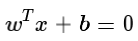其中，w 和 x 都是 n 维列向量，x 为平面上的点，w 为平面上的法向量，决定了超平面的方向，b 是一个实数，代表超平面到原点的距离。且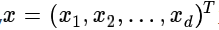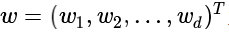那么，w 为什么是法向量呢？b 为什么表示平面到原点的距离呢？下面给出详细解释：

我们对“平面”概念的理解，一般是定义在三维空间中的，即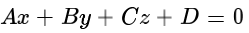这个平面由两个性质定义：1、方程是线性的，是由空间点的各分量的线性组合。2、方程数量是1。这个平面是建立在“三维”上的。如果我们撇开“维度”这个限制，那么就有了超平面的定义。实际上，超平面是纯粹的数学概念，不是物理概念，它是平面中的直线、空间中的平面的推广，只有当维度大于3，才称为“超”平面。它的本质是自由度比空间维度小1。自由度的概念可以简单的理解为至少要给定多少个分量的值才能确定一个点. 例如, 三维空间里的(超)平面只要给定了(x,y,z)中任意两个分量, 剩下的一个的值就确定了. 先确定值的两个分量是自由的, 因为它们想取什么值就能取什么值;剩下的那个是"不自由的", 因为它的值已经由另外两确定了. 二维空间里的超平面为一条直线. 一维空间里超平面为数轴上的一个点。

百度百科上对超平面的数学定义是这样的：超平面H是从n维空间到n-1维空间的一个映射子空间，它有一个n维向量和一个实数定义。因为是子空间，所以超平面一定过原点。

通常，R2（二维空间）中的点集 i = （x,y）满足等式 (点集 i 实际为一条直线)：

ax + 1/by + c = 0   (1)      (这里使用1/b 是为了后续计算好表示)

其中，a，b，c均为标量，a，1/b至少有一个不为0.我们假设 b 不为0。，那么

y = -abx  - cb

此时，使用换元法，令 t = x,（显然，t 为标量） 则点集 i (x,y) 可以表示成

i (x,y) =   ( t, -abt - cb） = t (1, -ab) + (0, -cb)

这条直线是什么？实际上就是过 (0, -cb) 点，方向为 (1, -ab) 的直线 L。

进一步，我们令向量 n  =  （a,1/b），则 （1）可以表示成n* i + c = 0  (2)

神奇的一刻来临了。假设在直线 L 上取一点 p0(x0，y0），显然，n* p0 + c = 0，那么 c = -n* p0.

更进一步，将 （2）改写，可得 n* i-n* p0 = 0 ，即可 n* （i - p0 ) = 0。

因为 n 和（i - p0 ) 均是向量，（i - p0 ) 在直线 L 上， 所以，n 垂直直线L ,即n为直线L 的法向量。更进一步，我们可以得到，那些与p的差向量与 n 向量正交的点，就是点集 i (x,y).

进一步解释什么是超平面：

给定向量空间 Rn 中的一个点 P 和一个非零向量n ,满足

n * （i - p）= 0

则称点集 i 为通过点p 的超平面，向量 n为通过超平面的法向量。按照这个定义，虽然当维度大于3才可以成为“超”平面，但是你仍然可以认为，一条直线是 R2 空间内的超平面，一个平面是 R3 空间内的超平面 。Rn 空间的超平面是Rn 空间内的一个 n - 1 维的仿射子空间。

点到超平面的距离

样本空间中的任意一点 x，到超平面（w,b）的距离，可以表示为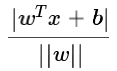后来有同学评论说，点到超平面上的点为什么这么计算呢？我在这里再具体说一下。推导过程并不繁琐（这里以三维空间为例）。

对于超平面A，假设 x‘ 为超平面上任意一点，那么，显然满足：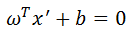对于空间上任意一点 x， 到平面 A  的距离 H，等于 x 到超平面的法向量长度，也就是 向量 xx' 在垂直方向上（即法向量）上的投影。而计算投影，将 xx' 乘以法向量 w 即可。并且，我们不光要投影，还要计算单位，即使用单位为 1 的投影。也就是在分母除以 || w ||。所以，距离 H 可以表示为：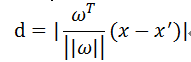又因为：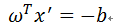所以，距离为：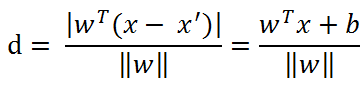判断超平面的正反

一个超平面可以将它所在的空间分为两半, 它的法向量指向的那一半对应的一面是它的正面, 另一面则是它的反面。如果利用数学来判断的话，需要利用到法向量 w。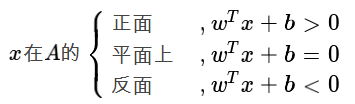若将距离公式中分子的绝对值去掉, 让它可以为正为负. 那么, 它的值正得越大, 代表点在平面的正向且与平面的距离越远. 反之, 它的值负得越大, 代表点在平面的反向且与平面的距离越远。
展开全文• 已知屏面上不重合的三点，如何求平面向量？...一个平面应该有两个方向上的法向量，Aireds所给出的答案法向量是指向哪个方向的？ 用定义做 任取其中两点成为一条直线 oa向量(a1,a2,a3


已知屏面上不重合的三点，如何求平面法向量？

已知平面上不重合的三点o(0,0,0)，a(a1,a2,a3)，b(b1,b2,b3)，那么此三点所确定平面的法向量n(x,y,z)中的x,y,z值分别是多少？谢谢！
一个平面应该有两个方向上的法向量，Aireds所给出的答案法向量是指向哪个方向的？

用定义做

任取其中两点成为一条直线
oa向量(a1,a2,a3) ob向量(b1,b2,b3)
(x,y,z)*(a1,a2,a3)=0
(x,y,z)*(b1,b2,b3)=0
解得((a2b3-a3b2)/(a3b1-a1b3)y,y,(a2b1-a1b2)/(a1b3-b1a3)y)
把y消掉通分得(a2b3-a3b2,a3b1-a1b3,a1b2-a2b1)
x=a2b3-a3b2,y=a3b1-a1b3,z=a1b2-a2b1


展开全文• 支持向量机SVM 前提概念 首先从字面的意思来看一下支持向量机，机即machine，也就是算法，那么什么是支持向量呢？支持向量支持的是什么呢？看一下以下几个概念 支撑超平面：设有一个集合C，x0C，x_0C，x0​为CCC...
• ## 空间直线与平面的交点

万次阅读 多人点赞 2011-08-15 12:45:40
如下图所示，已知直线L过点m（m1，m2，m3），且方向向量为VL（v1，v2，v3），平面P过点n（n1，n2，n3），且法线方向向量为VP（vp1，vp2，vp3），求得直线平面的交点O的坐标（x，y，z）： 将直线方程写成参数...float null
• 一路过去好像平面向量也是必学的知识,毕竟用初中知识解题的日子已经过去了.我们来看一个例题. Codeforces 935C Fifa and Fafa 这个题是求一个圆之中不覆盖某点(x,y)的最大圆的圆心坐标和半径.(在圆上算不覆盖) ...
• 在前两节中已经总结过了向量以及其运算，本篇内容开始...现在类推，有平面上一点坐标和该平面的法向量即可确定一个平面。 例题 例1 （二）平面的截距式方程 （二）平面的一般方程式 （三）两个平面的夹角 要注
• 还需要找到两类样本点之间离得最近的那一部分点（称之为支持向量），并将问题同样转化为找到中间一个超平面将这部分样本点划分开，此时又涉及到这些样本点到超平面的距离（即支持向量到超平面的距离），关于样本点到...
• ## 超平面的定义

千次阅读 2017-10-18 08:26:49
研究了半天，终于对“超平面”有了个初步了解。  n 维空间中的超平面由下面的...其中，w 和 x 都是 n 维列向量，x 为平面上的点，w 为平面上的法向量，决定了超平面方向，b 是一个实数，代表超平面到原点的距离。且
• 已知一个平面上的一点P0和法向量n，一条直线上的点L0和方向L,求该直线与该平面的交点P如下图首先我们分析一下我们知道平面直线的法向量，知道平面直线上的一点，求直线平面上的交点p。这里我们就要引入点积的...点乘
• 谁都能学会的SVM（支持向量机）分类器（一）超平面篇 机器学习领域问题实现模式一般为...wTx+b=0其中，w和x都是n维列向量，x 为平面上的点，w 为平面上的法向量，决定了超平面方向，b 是一个实数，代表超平面到原点的机器学习 人工智能
• ## 法向量

千次阅读 2018-10-18 15:17:15
向量是空间解析几何的一个概念，垂直于平面直线所表示的向量为该平面的法向量。由于空间内有无数个直线垂直于已知平面，因此一个平面都存在无数个法向量（包括两个单位法向量）。 定义 三维平面的法线是垂直于...
• 若 V是Rn 的一个子空间，已知V的一组基向量 {b1, b2, b3,... bk} 则： 可构建矩阵 ：A(nxk) = {b1 b2 b3 b4.....矩阵 A 包含平面的基向量 A(3x3) 根据投影的定义有 ： 原向量 - 投影向量 = 投影向量的正交补 ...
• 1、方向向量（direction vector）是一个数学概念，空间直线方向用一个与该直线平行的非零向量来表示，该向量称为这条直线的一个方向向量。 2、直线l⊥α，取直线l的方向向量a，则向量a叫做 法向量 平面α的法...
• http://blog.csdn.net/m0_37658225/article/details/70048959我们最常见的平面概念是在三维空间中定义的（这里用它做引申）: Ax+By+Cz+D=0 它由两个性质上定义: 1）方程是线性的: 是空间点的各分量的线性组合 2）...svm
• 1. 向量在另外一个向量上的投影 -以下是向量a在向量b上的投影，θ 为两向量的夹角 向量a在向量b上的投影的计算公式为： ...#vectorA为直线方向向量，vectorB为平面的法向量，则求投影方向vectorT vectorT=vectorB-n线性代数
• 综上：对矩阵A做奇异值分解，最小奇异值对应的特征向量就是拟合平面的系数向量。 根据一组点的坐标拟合空间平面，有两种方法 第一种：如果在测量得到的数据中，x，y值都是确认没有误差的，而误差只是出现在z值上...
• ## 向量

2015-02-07 01:17:14
平面向量 1、  数量 ： 只有大小的量  向量 ： 既有大小，又有方向的量 2、  有向线段 ： 确定了方向的线段。规定了起点与终点。->AB  三要素 ： 起点、方向、长度 3、  向量的表示  (1)、用有向线段...
• 如下图所示，已知直线L过点m（m1，m2，m3），且方向向量为VL（v1，v2，v3），平面P过点n（n1，n2，n3），且法线方向向量为VP（vp1，vp2，vp3），求得直线平面的交点O的坐标（x，y，z）： 将直线方程写成...
• 直线方向向量平面的法向量向量 定义 垂直于平面直线所表示的向量为该平面的法向量平面的法向量：ax+by+cz+d=0，向量(a,b,c)就是其法向量方向向量 定义 方向向量是平行于直线向量 (x-x0)/m=(y-y0...
• 高维几何是泛函研究的主要对象之一，可对何谓高维几何，笔者没见到明显的定义。笔者认为，所谓高维几何，就是把高维向量空间，同时视为点的集合，研究她的子集的性质和子集之间的关系的学科，就是高维几何(是否如此...
• ## 向量点乘与叉乘

万次阅读 多人点赞 2019-02-25 14:06:01
向量点乘与叉乘 向量（Vector）  在几乎所有的几何问题中，向量...这里大家要特别注意，我这样说并不代表向量定义了起点和终点。向量仅仅定义方向和长度。 向量加法  向量也支持各种数学运算。最简单的就是加法。...
• Hyperplane – from Wolfram MathWorlda1,a2,…,ana_1,a_2,\ldots,a_n 为一组不全为 0 的纯量，如下定义的集合 SS 由这样的向量构成，x=[x1,x2,…,xn]Tx=[x_1,x_2,\ldots,x_n]^T（x∈Rnx\in \mathbb R^n），需要满足...
• 在二维平面内，已知共点的多条线基本可以通过解析几何的方法将点求出。但是在计算机中，由于浮点数等计算误差，导致多条线中相交的点不在同一位置。另外，在现实情况中，测距...欧几里得空间中二维平面直线交点 ...matlab 空间几何...